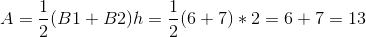## Example Questions

### Example Question #7 : How To Find The Area Of A Trapezoid

What is the area of this regular trapezoid?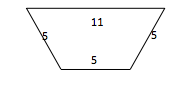26

45

20

32

32

Explanation:

To solve this question, you must divide the trapezoid into a rectangle and two right triangles. Using the Pythagorean Theorem, you would calculate the height of the triangle which is 4. The dimensions of the rectangle are 5 and 4, hence the area will be 20. The base of the triangle is 3 and the height of the triangle is 4. The area of one triangle is 6. Hence the total area will be 20+6+6=32. If you forget to split the shape into a rectangle and TWO triangles, or if you add the dimensions of the trapezoid, you could arrive at 26 as your answer.

### Example Question #1 : How To Find The Area Of A Trapezoid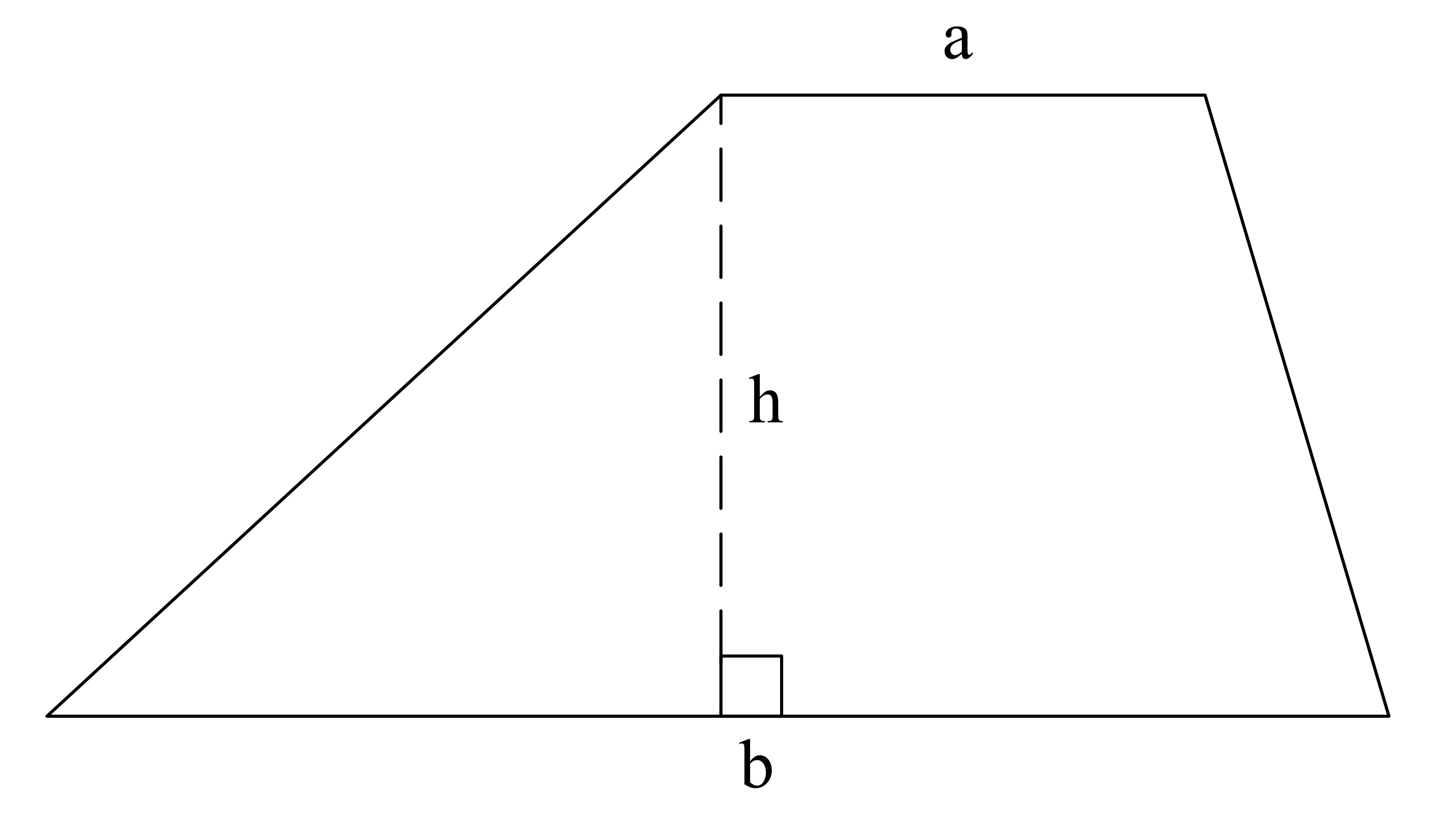What is the area of the trapezoid above if a = 2, b = 6, and h = 4?

16

32

24

64

8

16

Explanation:

Area of a Trapezoid = ½(a+b)*h

= ½ (2+6) * 4

= ½ (8) * 4

= 4 * 4 = 16

### Example Question #1 : How To Find The Area Of A Trapezoid

Find the area of a trapezoid if the height is, and the small and large bases areand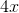, respectively.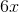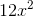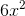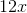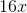Explanation:

Write the formula to find the area of a trapezoid.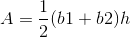Substitute the givens and evaluate the area.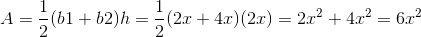### Example Question #2 : How To Find The Area Of A Trapezoid

Trapezoidhas an area of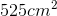. If height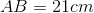and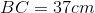, what is the measure of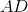?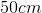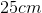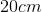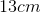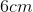Explanation:

The formula for the area of a trapezoid is: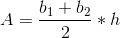We have here the height and one of the bases, plus the area, and we are being asked to find the length of base. Plug in known values and solve.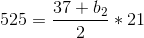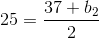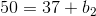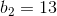Thus,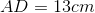### Example Question #3 : How To Find The Area Of A Trapezoid

Find the area of a trapezoid given bases of length 6 and 7 and height of 2.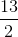Explanation:

To solve, simply use the formula for the area of a trapezoid.

Substitute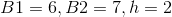into the area formula.

Thus,